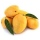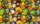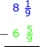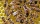# Fraction calculator

The calculator performs basic and advanced operations with fractions, expressions with fractions combined with integers, decimals, and mixed numbers. It also shows detailed step-by-step information about the fraction calculation procedure. Solve problems with two, three, or more fractions and numbers in one expression.

## Result:

### 3/4 - 3/10 = 9/20 = 0.45

Spelled result in words is nine twentieths.

### How do you solve fractions step by step?

1. Subtract: 3/4 - 3/10 = 3 · 5/4 · 5 - 3 · 2/10 · 2 = 15/20 - 6/20 = 15 - 6/20 = 9/20
For adding, subtracting, and comparing fractions, it is suitable to adjust both fractions to a common (equal, identical) denominator. The common denominator you can calculate as the least common multiple of both denominators - LCM(4, 10) = 20. In practice, it is enough to find the common denominator (not necessarily the lowest) by multiplying the denominators: 4 × 10 = 40. In the following intermediate step, it cannot further simplify the fraction result by canceling.
In other words - three quarters minus three tenths = nine twentieths.

#### Rules for expressions with fractions:

Fractions - simply use a forward slash between the numerator and denominator, i.e., for five-hundredths, enter 5/100. If you are using mixed numbers, be sure to leave a single space between the whole and fraction part.
The slash separates the numerator (number above a fraction line) and denominator (number below).

Mixed numerals (mixed fractions or mixed numbers) write as integer separated by one space and fraction i.e., 1 2/3 (having the same sign). An example of a negative mixed fraction: -5 1/2.
Because slash is both signs for fraction line and division, we recommended use colon (:) as the operator of division fractions i.e., 1/2 : 3.

Decimals (decimal numbers) enter with a decimal point . and they are automatically converted to fractions - i.e. 1.45.

The colon : and slash / is the symbol of division. Can be used to divide mixed numbers 1 2/3 : 4 3/8 or can be used for write complex fractions i.e. 1/2 : 1/3.
An asterisk * or × is the symbol for multiplication.
Plus + is addition, minus sign - is subtraction and ()[] is mathematical parentheses.
The exponentiation/power symbol is ^ - for example: (7/8-4/5)^2 = (7/8-4/5)2

#### Examples:

subtracting fractions: 2/3 - 1/2
multiplying fractions: 7/8 * 3/9
dividing Fractions: 1/2 : 3/4
exponentiation of fraction: 3/5^3
fractional exponents: 16 ^ 1/2
adding fractions and mixed numbers: 8/5 + 6 2/7
dividing integer and fraction: 5 ÷ 1/2
complex fractions: 5/8 : 2 2/3
decimal to fraction: 0.625
Fraction to Decimal: 1/4
Fraction to Percent: 1/8 %
comparing fractions: 1/4 2/3
multiplying a fraction by a whole number: 6 * 3/4
square root of a fraction: sqrt(1/16)
reducing or simplifying the fraction (simplification) - dividing the numerator and denominator of a fraction by the same non-zero number - equivalent fraction: 4/22
expression with brackets: 1/3 * (1/2 - 3 3/8)
compound fraction: 3/4 of 5/7
fractions multiple: 2/3 of 3/5
divide to find the quotient: 3/5 ÷ 2/3

The calculator follows well-known rules for order of operations. The most common mnemonics for remembering this order of operations are:
PEMDAS - Parentheses, Exponents, Multiplication, Division, Addition, Subtraction.
BEDMAS - Brackets, Exponents, Division, Multiplication, Addition, Subtraction
BODMAS - Brackets, Of or Order, Division, Multiplication, Addition, Subtraction.
GEMDAS - Grouping Symbols - brackets (){}, Exponents, Multiplication, Division, Addition, Subtraction.
Be careful, always do multiplication and division before addition and subtraction. Some operators (+ and -) and (* and /) has the same priority and then must evaluate from left to right.

## Fractions in word problems:

• Mother 7Mother bought 18 fruits. 1/3 were pineapple and the rest  were mangoes . how many were mangoes
• KevinKevin is baking brownies and needs peanuts.  The recipe calls for 1 and 2/3 cups of peanuts.  Kevin only has 5/6 of a cup of peanuts.  How many more cups of peanuts does Kevin need to have the correct amount?
• When 5When 5 is subtracted from both my numerator and denominator I become 3/4. What fraction i am?
• Equation with mixed 2A number, X, is subtracted from 8 1/4. The result is 12 3/5. What is the value of X?
• Mr. PeterMr. Peter mowed 2/7 of his lawn. His son mowed 1/4 of it. Who mowed the most? How much of the lawn still need to be mowed?
• KarinaKarina has 5 4/7 meters of cloth. She needs 3 1/6 meters of it in making a curtain for her window. How many meters of cloth were left to her after making the curtain?
• 5 2/55 2/5 hours a week  mathematics,  3 3/4 hours a week   Natural sciences, 4 3/8 hours a week  Technology . how many hours does  he spend on social sciences if he spend 17 1/2 hours a week for the four subject?
• There 14There are 250 people in a museum. 2/5 of the 250 people are girls 3/10 of the 250 people are boys The rest of the 250 people are adults Work out the number of adults in the museum.
• CandiesThere are 150 candies in the bowl. Ivan took 7 thirtieths, Charles 6 fifteenths, and Thomas 3 tenths. How many sweets are left in the bowl?
• Evaluate - lowest termsEvaluate: 16/25 - 11/25 (Express answer as a fraction reduced to lowest terms. )
• Mixed numbersFive and two-thirds minus 2 and one-half equals what number? A three and one-sixth B three and two-thirds C three and one-half D three and five-sixths
• Pizza fractionsAnn ate a third of a pizza and then another quater. Total part of pizza eaten by Ann and how much pizza is left?
• HoneyIla collected the honey from 3 of her beehives. From the first hive, she collected 2/3 gallons of honey. The last two hives yielded 1/4 gallon each. After using some of the honey she collected for baking, Lila found that she only had 3/4 gallon of honey l